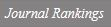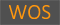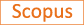Composition law and complex multiplication

Title
Composition law and complex multiplication
Authors
Eum, Ick SunJung, Ho YunKoo, Ja KyungShin, Dong Hwa
Ewha Authors
Issue Date
2020
Journal Title
JOURNAL OF NUMBER THEORY
ISSN
0022-314X1096-1658Citation
JOURNAL OF NUMBER THEORY vol. 209, pp. 396 - 420
Keywords
Binary quadratic formsClass field theoryComplex multiplicationModular functions
Publisher
Indexed
SCIE; SCOPUSDocument Type
Article
Abstract
Let K be an imaginary quadratic field of discriminant dK, and let n be a nontrivial integral ideal of K in which N is the smallest positive integer. Let Q(N) (d(K)) be the set of primitive positive definite binary quadratic forms of discriminant d(K) whose leading coefficients are relatively prime to N. We adopt an equivalence relation similar to(n) on Q(N) (dK) so that the set of equivalence classes Q(N)(d(K))/ similar to(n) can be regarded as a group isomorphic to the ray class group of K modulo n. We further establish an explicit isomorphism of Q(N)(d(K))/ similar to(n) onto Gal(K-n/K) in terms of Fricke invariants, where K-n denotes the ray class field of K modulo n. This would be a certain extension of the classical composition theory of binary quadratic forms, originated and developed by Gauss and Dirichlet. (C) 2019 Elsevier Inc. All rights reserved.
DOI
10.1016/j.jnt.2019.09.005
Appears in Collections:
연구기관 > 수리과학연구소 > Journal papers
Files in This Item:
There are no files associated with this item.
Export
RIS (EndNote)
XLS (Excel)
XML